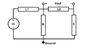# Solving a Peculiar Circuit: Find the Transfer Function

• Engineering
• Leomusic
In summary, the conversation discussed a circuit with a peculiar transfer function where Vout is referenced across an inductor instead of ground. The homework problem was to find the transfer function Vout(s)/Vi(s) using the given values for R, L1, L2, and C. The participants discussed using the mesh-current method to solve for the loop currents and obtaining the desired transfer function. Nodal analysis was also mentioned as a viable method. The final consensus was that the solution provided by Leo was correct and there may be simpler methods for solving this type of problem.

#### Leomusic

Hi all,

New to the forums here, but I've got a question for you guys:

I've ran into this rather peculiar circuit. In all the circuits I've seen (as far as transfer functions go), Vout has always referenced ground (e.g. one node of Vout is ground); in this one, however Vout is across an inductor. I've attached the circuit diagram. (excuse the crudeness of the circuit diagram...quickly whipped it up on Paint).

## Homework Statement

Find the transfer function Vout(s)/Vi(s) of the circuit.

R=1Ω, L1=2H, L2=3H, C=0.1F

## Homework Equations

Zr = R; Zl=Ls; Zc=1/Cs; KVL; KCL, etc.

## The Attempt at a Solution

Since Vout wasn't referencing ground, I figured that I needed to use the mesh-current method to get equations for Vi and Vout for each loop. After some rather nasty symbolic matrix algebra, I got expressions for I1 and I2 (loop currents), plugged each of those back into the mesh-current equations, and obtained:

$H(s)=\frac{V_{out}}{V_{i}}=\frac{3s^2}{6s^3+5s^2+20s+10}$

Is this correct? Also another question: is there a simpler way to do this? Forgive my idiocy but I might be missing something terribly obvious...

It's been a while since this textbook stuff! I tinker around quite a bit with circuits, but I'm no EE...I guess that's what I get for being mechanicalThanks everyone!

-Leo.

#### Attachments

•RLC problem.png
6.9 KB · Views: 557
Last edited:

Leomusic said:
Is this correct? Also another question: is there a simpler way to do this? Forgive my idiocy but I might be missing something terribly obvious...

Nodal analysis also works well for these transfer function problems. See the image.

#### Attachments

Sounds good! Thanks a lot guys.

-Leo.